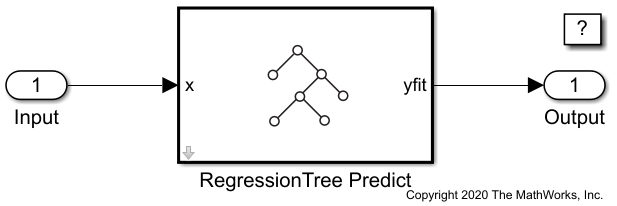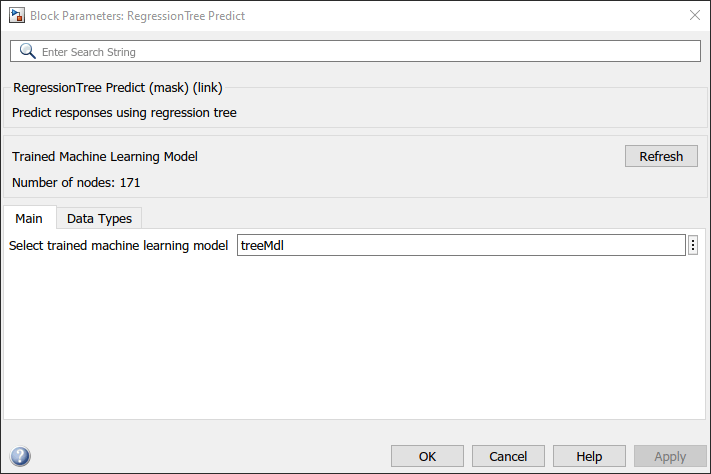# Predict Responses Using RegressionTree Predict Block

This example shows how to use the RegressionTree Predict block for response prediction in Simulink®. The block accepts an observation (predictor data) and returns the predicted response for the observation using the trained regression tree model.

### Train Regression Model

Load the `carbig` data set, which contains measurements of cars made in the 1970s and early 1980s. Create a matrix containing the predictor variables and a vector of the response variable.

```load carbig X = [Acceleration,Cylinders,Displacement,Horsepower,Model_Year,Weight]; Y = MPG;```

Train a regression tree model.

`treeMdl = fitrtree(X,Y);`

`treeMdl` is a `RegressionTree` model.

This example provides the Simulink model `slexCarDataRegressionTreePredictExample.slx`, which includes the RegressionTree Predict block. You can open the Simulink model or create a new model as described in this section.

Open the Simulink model `slexCarDataRegressionTreePredictExample.slx`.

```SimMdlName = 'slexCarDataRegressionTreePredictExample'; open_system(SimMdlName)```If you open the Simulink model, then the software runs the code in the `PreLoadFcn` callback function before loading the Simulink model. The `PreLoadFcn` callback function of `slexCarDataRegressionTreePredictExample` includes code to check if your workspace contains the `treeMdl` variable for the trained model. If the workspace does not contain the variable, `PreLoadFcn` loads the sample data, trains the tree model, and creates an input signal for the Simulink model. To view the callback function, in the Setup section on the Modeling tab, click Model Settings and select Model Properties. Then, on the Callbacks tab, select the `PreLoadFcn` callback function in the Model callbacks pane.

To create a new Simulink model, open the Blank Model template and add the RegressionTree Predict block. Add the Inport and Outport blocks and connect them to the RegressionTree Predict block.

Double-click the RegressionTree Predict block to open the Block Parameters dialog box. You can specify the name of a workspace variable that contains the trained tree model. The default variable name is `treeMdl`. Click the Refresh button. The dialog box displays the options used to train the tree model `treeMdl` under Trained Machine Learning Model.The RegressionTree Predict block expects an observation containing 6 predictor values. Double-click the Inport block, and set the Port dimensions to 6 on the Signal Attributes tab.

Create an input signal in the form of a structure array for the Simulink model. The structure array must contain these fields:

• `time` — The points in time at which the observations enter the model. The orientation must correspond to the observations in the predictor data. So, in this example, `time` must be a column vector.

• `signals` — A 1-by-1 structure array describing the input data and containing the fields `values` and `dimensions`, where `values` is a matrix of predictor data, and `dimensions` is the number of predictor variables.

Create an appropriate structure array for the `slexCarDataRegressionTreePredictExample` model from the `carsmall` data set.

```load carsmall testX = [Acceleration,Cylinders,Displacement,Horsepower,Model_Year,Weight]; testX = rmmissing(testX); carsmallInput.time = (0:size(testX,1)-1)'; carsmallInput.signals(1).values = testX; carsmallInput.signals(1).dimensions = size(testX,2);```

To import signal data from the workspace:

• Open the Configuration Parameters dialog box. On the Modeling tab, click Model Settings.

• In the Data Import/Export pane, select the Input check box and enter `carsmallInput` in the adjacent text box.

• In the Solver pane, under Simulation time, set Stop time to `carsmallInput.time(end)`. Under Solver selection, set Type to `Fixed-step`, and set Solver to `discrete (no continuous states)`.

`sim(SimMdlName);`